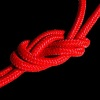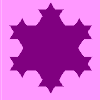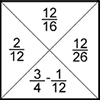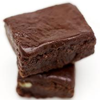Search by Topic

Resources tagged with Calculating with fractions similar to Rough Rectangle:

Filter by: Content type:
Age range:
Challenge level:

There are 36 results

Broad Topics > Fractions, Decimals, Percentages, Ratio and Proportion > Calculating with fractionsArchimedes and Numerical Roots

Age 14 to 16 Challenge Level:

The problem is how did Archimedes calculate the lengths of the sides of the polygons which needed him to be able to calculate square roots?Not Continued Fractions

Age 14 to 18 Challenge Level:

Which rational numbers cannot be written in the form x + 1/(y + 1/z) where x, y and z are integers?All Tangled Up

Age 14 to 18 Challenge Level:

Can you tangle yourself up and reach any fraction?Twisting and Turning

Age 11 to 14 Challenge Level:

Take a look at the video and try to find a sequence of moves that will untangle the ropes.Keep it Simple

Age 11 to 14 Challenge Level:

Can all unit fractions be written as the sum of two unit fractions?Harmonic Triangle

Age 14 to 16 Challenge Level:

Can you see how to build a harmonic triangle? Can you work out the next two rows?More Twisting and Turning

Age 11 to 16 Challenge Level:

It would be nice to have a strategy for disentangling any tangled ropes...Fracmax

Age 14 to 16 Challenge Level:

Find the maximum value of 1/p + 1/q + 1/r where this sum is less than 1 and p, q, and r are positive integers.Special Sums and Products

Age 11 to 14 Challenge Level:

Find some examples of pairs of numbers such that their sum is a factor of their product. eg. 4 + 12 = 16 and 4 × 12 = 48 and 16 is a factor of 48.Egyptian Fractions

Age 11 to 14 Challenge Level:

The Egyptians expressed all fractions as the sum of different unit fractions. Here is a chance to explore how they could have written different fractions.Sum Equals Product

Age 11 to 14 Challenge Level:

The sum of the numbers 4 and 1 [1/3] is the same as the product of 4 and 1 [1/3]; that is to say 4 + 1 [1/3] = 4 � 1 [1/3]. What other numbers have the sum equal to the product and can this be so for. . . .Unit Fractions

Age 11 to 14 Challenge Level:

Consider the equation 1/a + 1/b + 1/c = 1 where a, b and c are natural numbers and 0 < a < b < c. Prove that there is only one set of values which satisfy this equation.Lower Bound

Age 14 to 16 Challenge Level:

What would you get if you continued this sequence of fraction sums? 1/2 + 2/1 = 2/3 + 3/2 = 3/4 + 4/3 =There's a Limit

Age 14 to 18 Challenge Level:

Explore the continued fraction: 2+3/(2+3/(2+3/2+...)) What do you notice when successive terms are taken? What happens to the terms if the fraction goes on indefinitely?Fair Shares?

Age 14 to 16 Challenge Level:

A mother wants to share a sum of money by giving each of her children in turn a lump sum plus a fraction of the remainder. How can she do this in order to share the money out equally?Diminishing Returns

Age 11 to 14 Challenge Level:

How much of the square is coloured blue? How will the pattern continue?Countdown Fractions

Age 11 to 16 Challenge Level:

Here is a chance to play a fractions version of the classic Countdown Game.The Greedy Algorithm

Age 11 to 14 Challenge Level:

The Egyptians expressed all fractions as the sum of different unit fractions. The Greedy Algorithm might provide us with an efficient way of doing this.Tweedle Dum and Tweedle Dee

Age 11 to 14 Challenge Level:

Two brothers were left some money, amounting to an exact number of pounds, to divide between them. DEE undertook the division. "But your heap is larger than mine!" cried DUM...A Chance to Win?

Age 11 to 14 Challenge Level:

Imagine you were given the chance to win some money... and imagine you had nothing to lose...Hello Again

Age 11 to 14 Challenge Level:

Anne completes a circuit around a circular track in 40 seconds. Brenda runs in the opposite direction and meets Anne every 15 seconds. How long does it take Brenda to run around the track?Hands Together

Age 11 to 14 Challenge Level:

Sometime during every hour the minute hand lies directly above the hour hand. At what time between 4 and 5 o'clock does this happen?Smaller and Smaller

Age 7 to 14 Challenge Level:

Can you predict, without drawing, what the perimeter of the next shape in this pattern will be if we continue drawing them in the same way?Blue and White

Age 11 to 14 Challenge Level:

Identical squares of side one unit contain some circles shaded blue. In which of the four examples is the shaded area greatest?Age 11 to 16 Challenge Level:

The items in the shopping basket add and multiply to give the same amount. What could their prices be?Mathematical Swimmer

Age 11 to 14 Challenge Level:

Twice a week I go swimming and swim the same number of lengths of the pool each time. As I swim, I count the lengths I've done so far, and make it into a fraction of the whole number of lengths I. . . .Age 11 to 14 Challenge Level:

Aisha's division and subtraction calculations both gave the same answer! Can you find some more examples?Ben's Game

Age 11 to 14 Challenge Level:

Ben passed a third of his counters to Jack, Jack passed a quarter of his counters to Emma and Emma passed a fifth of her counters to Ben. After this they all had the same number of counters.Turning Tangles

Age 11 to 14 Challenge Level:

Look carefully at the video of a tangle and explain what's happening.Peaches Today, Peaches Tomorrow...

Age 11 to 14 Challenge Level:

Whenever a monkey has peaches, he always keeps a fraction of them each day, gives the rest away, and then eats one. How long could he make his peaches last for?Investigating the Dilution Series

Age 14 to 16 Challenge Level:

Which dilutions can you make using only 10ml pipettes?3388

Age 11 to 14 Challenge Level:

Using some or all of the operations of addition, subtraction, multiplication and division and using the digits 3, 3, 8 and 8 each once and only once make an expression equal to 24.Age 11 to 14 Challenge Level:

Can you work out which drink has the stronger flavour?Ratios and Dilutions

Age 14 to 16 Challenge Level:

Scientists often require solutions which are diluted to a particular concentration. In this problem, you can explore the mathematics of simple dilutionsFractions Jigsaw

Age 11 to 14 Challenge Level:

A jigsaw where pieces only go together if the fractions are equivalent.Tray Bake

Age 11 to 14 Challenge Level:

My recipe is for 12 cakes - how do I change it if I want to make a different number of cakes?